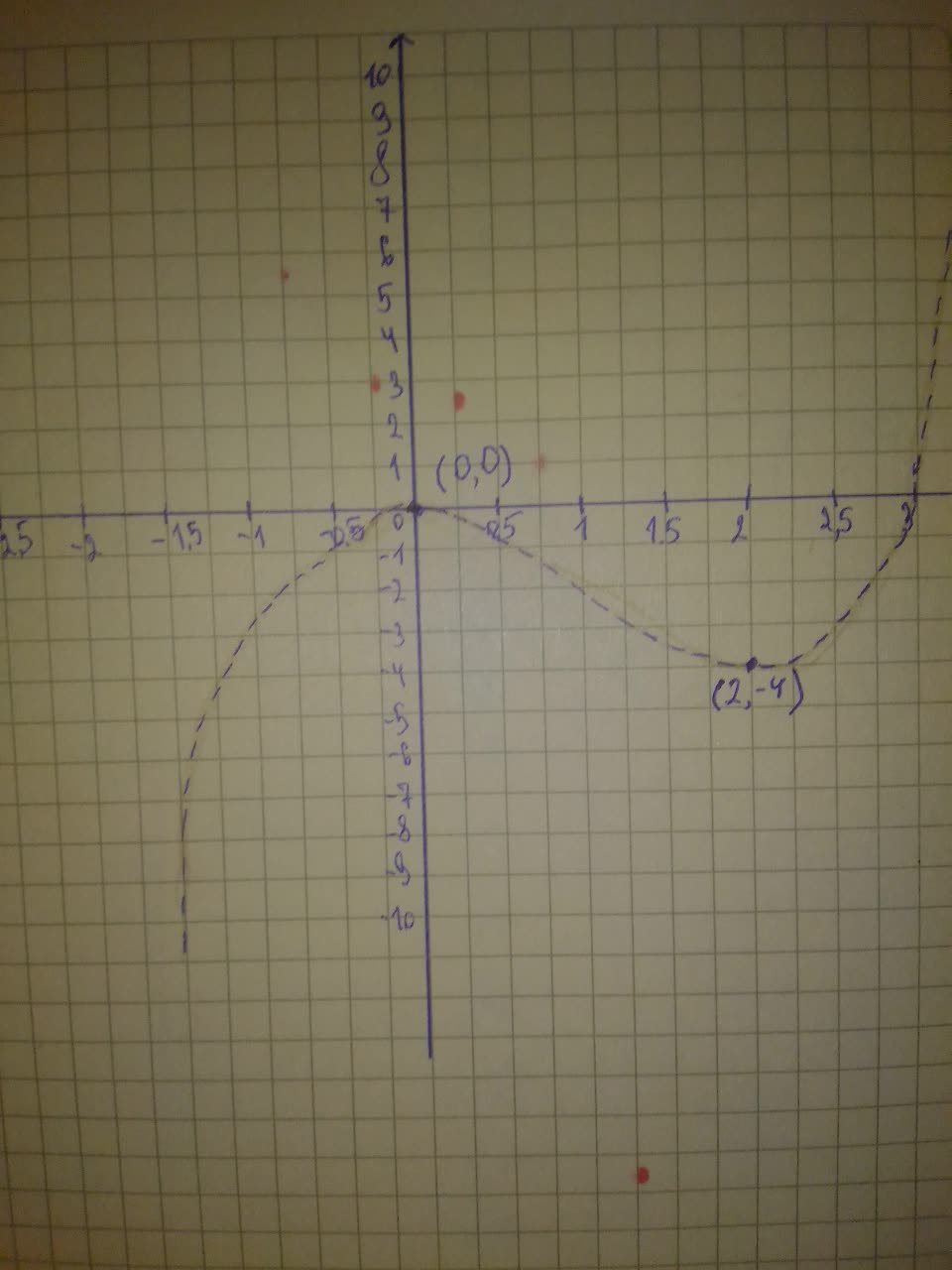# Graph the polynomial in the given viewing rectangle. Find the coordinates of all local extrema. State each answer rounded to two decimal places. State the domain and range. y=x^{3}-3x^{2},[-2,5] by [-10,10]Chaya Galloway 2021-02-20 Answered
Graph the polynomial in the given viewing rectangle. Find the coordinates of all local extrema. State each answer rounded to two decimal places. State the domain and range. $y={x}^{3}-3{x}^{2},\left[-2,5\right]by\left[-10,10\right]$
You can still ask an expert for help

• Live experts 24/7
• Questions are typically answered in as fast as 30 minutes
• Personalized clear answers

Solve your problem for the price of one coffee

• Math expert for every subject
• Pay only if we can solve itAnonym

Step 1
$y={x}^{3}-3{x}^{2},\left[-2,5\right]×\left[-10,10\right]$
The graph of this function in the indicated viewing window is shown in the following picture.Step 2
As we can see in this picture this function has:
A local maximum that occurs at the point (0, 0).
A local minimum that occurs at the point (2,-4).
For the domain:
This is a polynomial function so it is defined for all values of x in the set of real numbers.
The domain is: $D=\left(-\mathrm{\infty },+\mathrm{\infty }\right)$
For the range:
This function can get all values in the set of real numbers (vertical axis) so:
The range is: $R=\left(-\mathrm{\infty },+\mathrm{\infty }\right)$

We have step-by-step solutions for your answer!